# Electronics and Communication Engineering - Electromagnetic Field Theory

6.

The input impedance of short-circuited line of length l where λ/4 < l < λ/2, is

 A. resistive B. inductive C. capacitive D. none of the above

Explanation:

l < λ/4, ZL = 0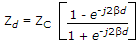, if Zd comes +j kZC then inductive,

If Zd comes -j kZC then capacitive.

for 0 ≤ l ≤ 1/4, λ ≤ l ≤ 3λ/4 will be inductive.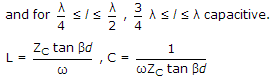7.

A wave is propagated in a waveguide at frequency of 9 GHz and separation is 2 cm between walls find cut off wavelength for dominant mode.

 A. 4 cm B. 1 cm C. 2 cm D. 8 cm

Explanation: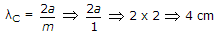.

8.

Find the radiation resistance of an antenna of length λ/10 meter?

 A. 8 Ω B. 780 Ω C. 7.8 Ω D. 78 Ω

Explanation: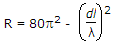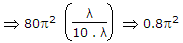.

9.

Charge needed within a unit sphere centred at the origin for producing a potential field,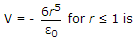A. 12 pc B. 60 pc C. 120 pc D. 180 pc

Explanation: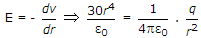q = 120p coulomb, r = 1 meter.

10.

A rectangular metal waveguide filled with a dielectric of relative permittivity εr = 4, has the inside dimensions 3 x 1.2 cm, the cut off frequency for the dominant mode is

 A. 2.5 GHz B. 5 GHz C. 10 GHz D. 12.5 GHz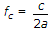for dominant mode.